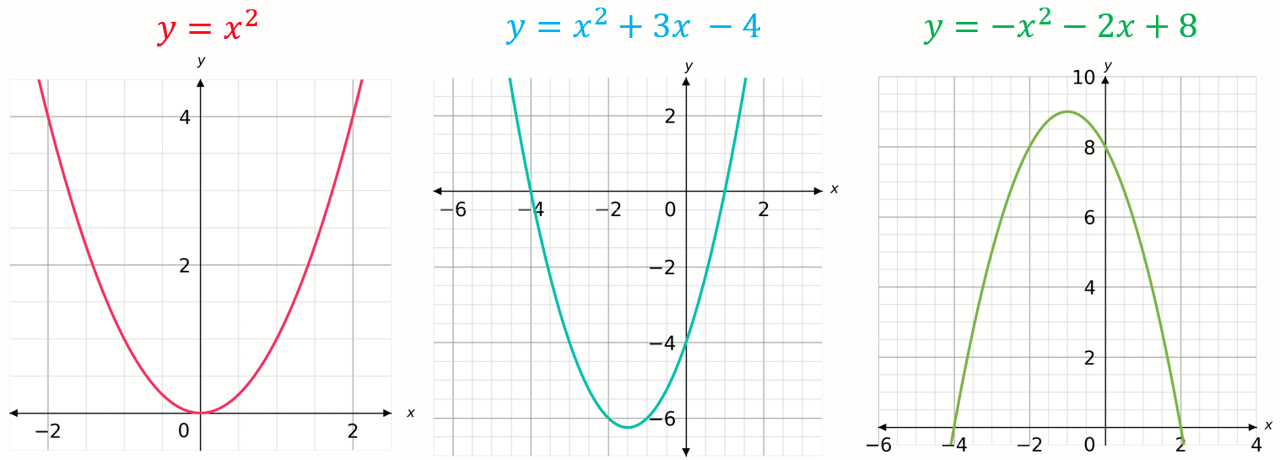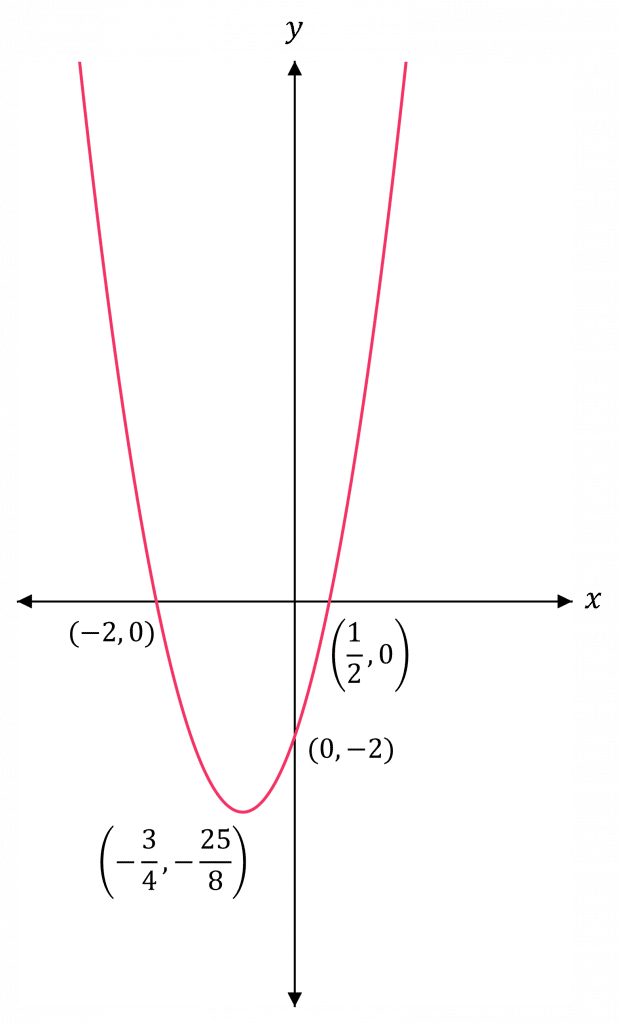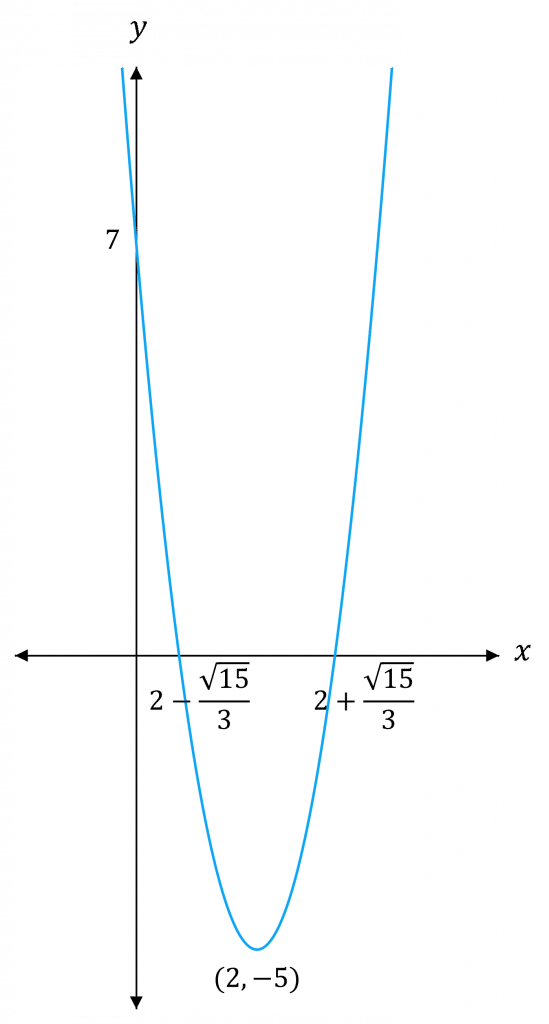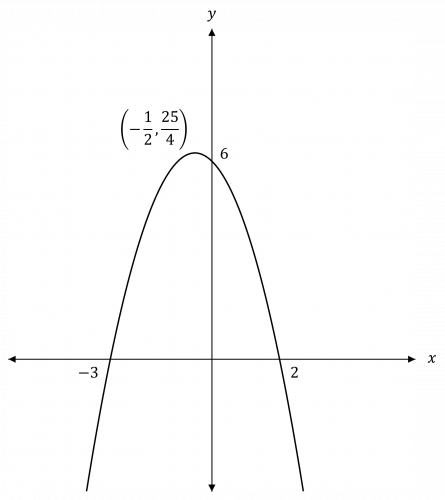A LevelAQAEdexcelOCR

You will need to be able to identify and sketch quadratic graphs.

Make sure you are happy with the following topics before continuing.

A LevelAQAEdexcelOCR

Quadratic graphs have the general form

$\textcolor{red}{a} x^2 + \textcolor{blue}{b}x + \textcolor{limegreen}{c}$

These can be either u-shaped (if $\textcolor{red}{a}$ is positive) or n-shaped (if $\textcolor{red}{a}$ is negative)

Some examples of quadratic graphs can bee seen below:A LevelAQAEdexcelOCR

Step 1: Decide whether the graph is an n-shape or a u-shape.

• u-shaped if the coefficient of $x^2$ is positive
• n-shaped if the coefficient of $x^2$ is negative

Step 2: Find the intersections with the $x$-axis (set $y=0$) and $y$-axis (set $x=0$)

Step 3: Find the maximum (if it is an n-shaped graph) or minimum (if it is a u-shaped graph) using symmetry or completing the square.

Step 4: Sketch the graph and label all the important points.

Example: Sketch the graph of $y = 2x^2 + 3x - 2$Step 1: The coefficient of $x^2$ is positive, so the graph is u-shaped.

Step 2: When $y=0$,

\begin{aligned} 2x^2 + 3x - 2 &= 0 \\ (2x-1)(x+2) &= 0 \end{aligned}

$x = \dfrac{1}{2}$  or  $x = -2$

Hence, the curve crosses the $x$-axis at $\textcolor{red}{\left( \dfrac{1}{2}, 0 \right)}$ and $\textcolor{red}{(-2,0)}$

When $x = 0$,

$y = 2(0)^2 + 3(0) - 2 = -2$

Hence, the curve crosses the $y$-axis at $\textcolor{red}{(0,-2)}$

Note: You can often read off the value the graph crosses the $y$-axis at (called the $y$-intercept) from the equation of a quadratic. The $y$-intercept of $y=ax^{2}+bx+c$ is always $c$.

Step 3: The minimum value will be halfway between $\left(\dfrac{1}{2}, 0 \right)$ and $(-2,0)$:

$\left( \dfrac{1}{2} - 2 \right) \div 2 = - \dfrac{3}{4}$

So, the minimum value is at $x = - \dfrac{3}{4}$

Therefore, the minimum is

$y = 2 \left(- \dfrac{3}{4} \right)^2 + 3 \left(- \dfrac{3}{4} \right) - 2 = - \dfrac{25}{8}$

So, the graph has a minimum at the point $\textcolor{red}{\left(- \dfrac{3}{4}, - \dfrac{25}{8} \right)}$

Step 4: Sketch the graph (sketching doesn’t need to be to scale) and label all the important points.

A LevelAQAEdexcelOCR

## Completing the Square to Sketch Graphs

You can complete the square to help sketch quadratic curves.

Example: Sketch the curve of $y = 3x^2 - 12x + 7$Firstly, complete the square:

$y = 3x^2 - 12x + 7 = 3(x-2)^2 - 5$

When $y=0$,

\begin{aligned} 3(x-2)^2 - 5 &=0 \\ (x-2)^2 &= \dfrac{5}{3} \\ x - 2 &= \pm \sqrt{\dfrac{5}{3}} \\ x &= 2 \pm \dfrac{\sqrt{15}}{3} \end{aligned}

So, the curve crosses the $x$-axis at $\textcolor{red}{\left(2-\dfrac{\sqrt{15}}{3},0 \right)}$ and $\textcolor{red}{\left(2+\dfrac{\sqrt{15}}{3},0 \right)}$

When $x=0$,

$y = 3(0)^2 - 12(0) + 7 = 7$

So, the curve crosses the $y$-axis at $\textcolor{red}{(0,7)}$

$(x-2)^2 \geq 0$ (since a square number is never less than $0$), therefore the minimum value occurs at $x-2=0 \Rightarrow x = 2$, and therefore the minimum is

$y = 3(2-2)^2 - 5$

So, the minimum is at the point $\textcolor{red}{(2, -5)}$

Then, you have enough information to sketch the graph.

Note: You can read off the vertex of a graph from completed square form. The vertex of $y=a(x+b)^{2}+c$ is always at $(-b,c)$.

A LevelAQAEdexcelOCR

## Functions with no Real Roots

Some functions have no real roots – i.e. its graph doesn’t cross the $x$-axis. You can complete the square and use this to see if quadratics have real roots, or not.

Example: $f(x) = x^2 + 2x + 6$. Determine whether $f(x)$ has any real roots.

Complete the square:

$f(x) = x^2 + 2x + 6 = \textcolor{red}{(x+1)^2} + \textcolor{blue}{5}$

$\textcolor{red}{(x+1)^2}$ is never less than $0$, therefore the minimum of the curve of $f(x)$ is at $\textcolor{blue}{5}$.

Hence, $f(x)$ cannot be negative and will not cross the $x$-axis at any point.

Therefore, $f(x)$ has no real roots.

Note: If the coefficient of $x^2$ is negative, you can do a similar thing to see if $f(x)$ has real roots, by checking if $f(x)$ is ever positive.

A LevelAQAEdexcelOCR

The coefficient of $x^2$ is negative, so the graph is n-shaped.

When $x = 0$, $y = 6$

When $y = 0$,

\begin{aligned} 6 - x - x^2 &= 0 \\ (2-x)(x+3) &= 0 \end{aligned}

$x = 2$ or $x = -3$

The maximum value is halfway between $-3$ and $2$. So the maximum is at $x = - \dfrac{1}{2}$ and $y = 6 - \left(- \dfrac{1}{2} \right) - \left(- \dfrac{1}{2} \right)^2 = \dfrac{25}{4}$

So, we have enough information to plot the graph:The coefficient of $x^2$ is positive, so there will be a minimum.

\begin{aligned} f(x) &= 2x^2 + 16x - 10 \\ &= 2 \left(x+\dfrac{16}{2 \times 2} \right)^2 + \left(-10 - 2 \left(\dfrac{16}{2 \times 2}\right)^2 \right) \\ &= 2(x+4)^2 - 42 \end{aligned}

Hence, the minimum occurs at $(x+4) = 0 \Rightarrow x = -4$

So, the minimum is

$f(-4) = 2(-4+4)^2 - 42 = -42$

Hence, the graph has a minimum at the point $(-4, -42)$

\begin{aligned} f(x) &= -2x^2 - 8x - 12 \\ &= -(2x^2 + 8x + 12) \end{aligned}

Complete the square:

$2x^2 + 8x + 12 \\ = 2 \left(x+\dfrac{8}{2 \times 2} \right)^2 + \left(12 - 2\left(\dfrac{8}{2 \times 2}\right)^2 \right) \\ = 2(x+2)^2 + 4$

So, $f(x) = - 2(x+2)^2 - 4$

$(x+2)^2$ is never less than $0$, therefore the maximum of the curve of $f(x)$ is at $-4$.

Hence, $f(x)$ cannot be positive and will not cross the $x$-axis at any point.

Therefore, the function has no real roots.

A Level

A Level

A Level

A Level

## You May Also Like...### MME Learning Portal

Online exams, practice questions and revision videos for every GCSE level 9-1 topic! No fees, no trial period, just totally free access to the UK’s best GCSE maths revision platform.

£0.00